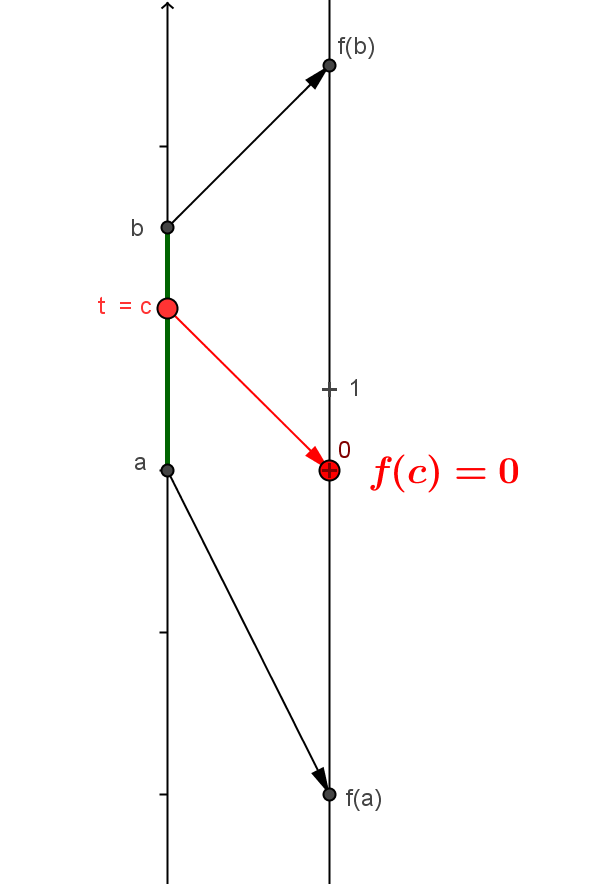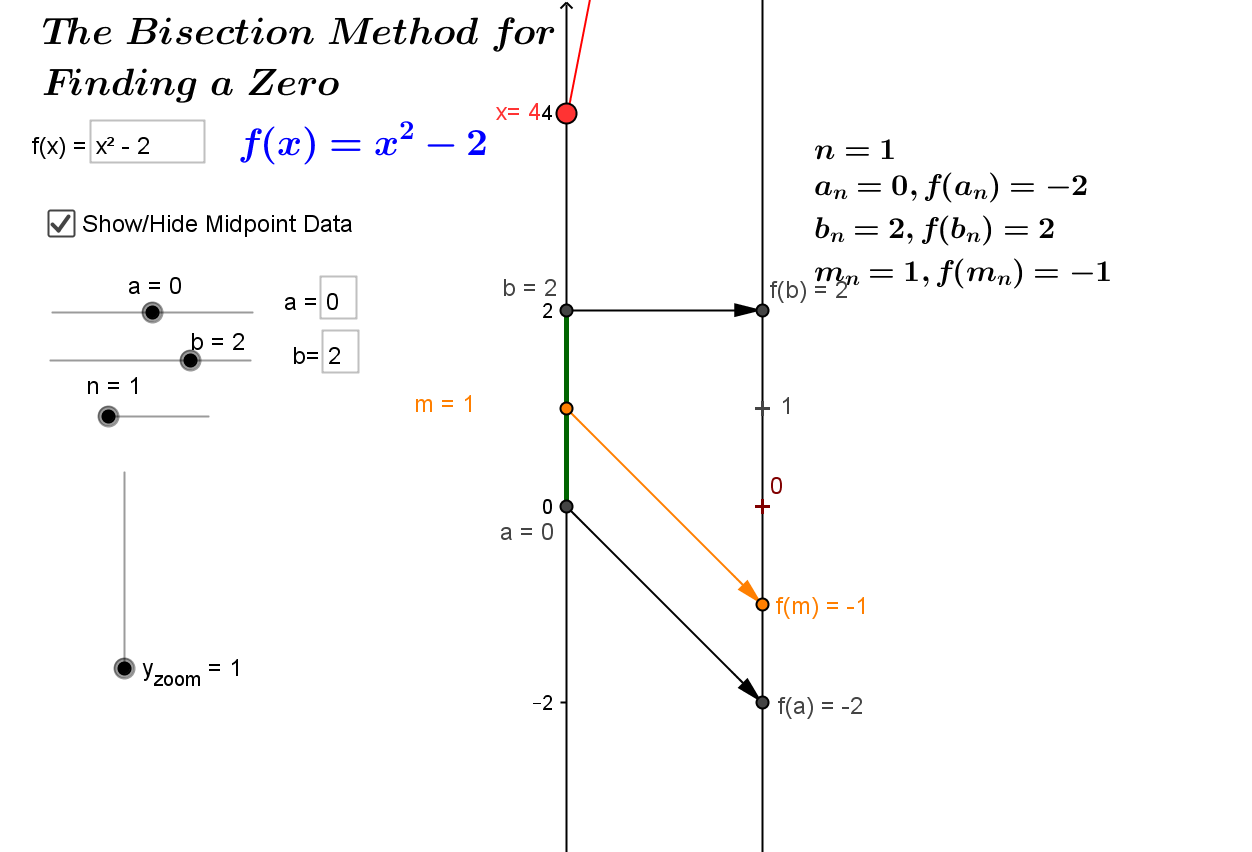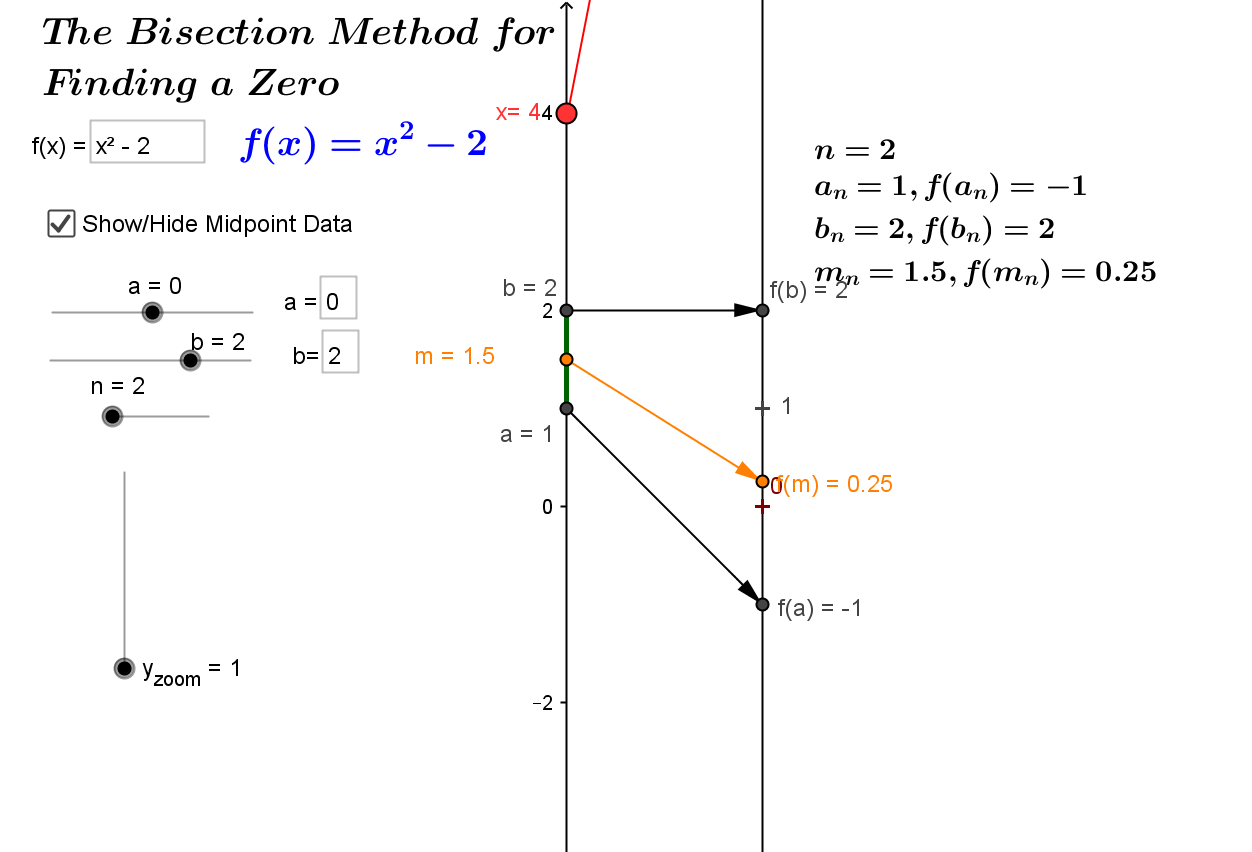Theorem: CCD.IVT.0: If $f$ is a continuous function on the interval $[a,b]$ and $0$ is between $f(a)$ and $f(b)$ or $f(a) \cdot f(b) < 0$, then there is a number $c \in [a,b]$  where  $f(c) = 0$. Theorem: CCD.IVT: If $f$ is a continuous function on the interval $[a,b]$ and $v$ is between $f(a)$ and $f(b)$, then there is a number $c \in [a,b]$  where  $f(c) = v$ For the mapping diagram visualization of IVT.0, the continuity of the function $f$ can be interpreted as the function representing the position of an object at varying times between times $a$ and $b$. The assumption that  $0$ is between $f(a)$ and $f(b)$ is interpreted that between times $a$ and $b$ the object has moved either from a negative to a positive position or vice versa.The conclusion is interpreted that at some time, $c$, the object's position is precisely $0$, i.e., $f(c)=0$. Not only do mapping diagrams provide a distinct alternative to the graphical visualization of the intermediate value theorem, they also provide a sensible alternative visualization of the proof of IVT.0 by the Bisection Method.Mapping Diagram for IVT.0 Proof Outline and Visualization of CCD.IVT.0 using the "bisection method."Suppose $f$ is a continuous function on the interval $[a,b]$ and  $f(a) \cdot f(b) < 0$, then let $a_1=a, b_1=b$ and  $c_1 =\frac {a_1+b_2}2$ the midpoint of the interval $[a_1,b_1]$.
If $f(c_1)=0$, the let $c=c_1$ and the theorem is proven.
If  $f(a_1)\cdot f(c_1) >0 then let$a_2 = a_1$and$b_2=c_1$. Otherwise let$a_2 = c_1$and$b_2=b_1$. In either case$f(a_2) \cdot f(b_2) < 0$and the procedure can be continued. At each step$b_{n+1}-a_{n+1} = \frac {b_n-a_n}2$so the sequences$\{a_n\}$and$\{b_n\}$with$f(a_n) \cdot f(b_n) < 0$approach a single number$c \in[a,b]$and by continuity$f(c) = 0\$.

The Bisection Method and IVT visualized with GeoGebra.